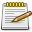# Difference between revisions of "Vector/Vector2"

Jump to navigation Jump to search

The Vector2 class is a class introduced in 1.4

## Methods

### create

This is default constructor for the Vector2 class and returns a Vector2 object.

#### Syntax

`vector2 Vector2 ( float x = 0, float y = 0 )`
• x, and y coordinates for the vector. If not specified, they default to 0.
• Instead of these two coordinates, a single Vector2 object may be inserted to clone it.

#### Example

This example ...

Click to collapse [-]
SharedScript Example Missing Function Vector/Vector2 needs a script example, help out by writing one. Before submitting check out Editing Guidelines Script Examples.
```-- TODO
```

### getX/Y and setX/Y

These functions allow you get and set specific coordinates:

• getX and setX
• getY and setY

#### Generic set syntax

`bool vec:setX ( float x = 0 )`
• x: number value to set the coordinate to
• This value can also be set by the variable vec.x
• Returns true if doesn't fail

#### Generic get syntax

`float vec:getX()`
• This value can also be accessed by the variable vec.x
• Returns a float if doesn't fail